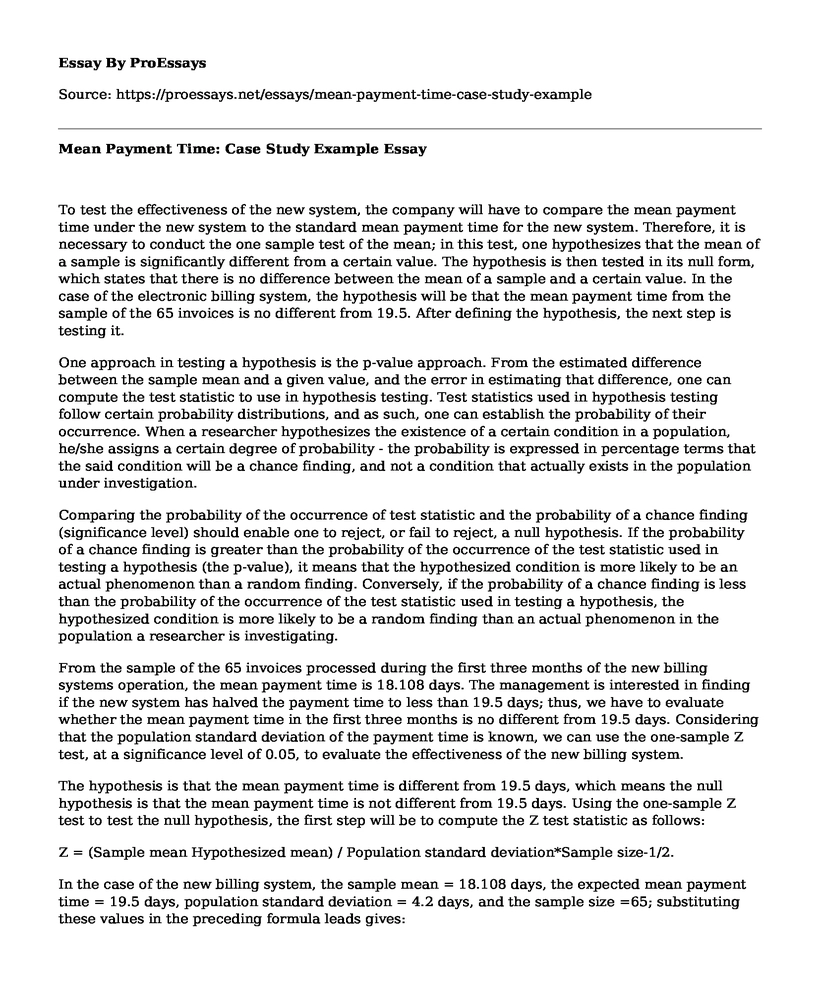# Mean Payment Time: Case Study Example

 Paper Type:  Case study Pages:  3 Wordcount:  608 Words Date:  2021-06-18
Categories:

To test the effectiveness of the new system, the company will have to compare the mean payment time under the new system to the standard mean payment time for the new system. Therefore, it is necessary to conduct the one sample test of the mean; in this test, one hypothesizes that the mean of a sample is significantly different from a certain value. The hypothesis is then tested in its null form, which states that there is no difference between the mean of a sample and a certain value. In the case of the electronic billing system, the hypothesis will be that the mean payment time from the sample of the 65 invoices is no different from 19.5. After defining the hypothesis, the next step is testing it.

Is your time best spent reading someone else’s essay? Get a 100% original essay FROM A CERTIFIED WRITER!

One approach in testing a hypothesis is the p-value approach. From the estimated difference between the sample mean and a given value, and the error in estimating that difference, one can compute the test statistic to use in hypothesis testing. Test statistics used in hypothesis testing follow certain probability distributions, and as such, one can establish the probability of their occurrence. When a researcher hypothesizes the existence of a certain condition in a population, he/she assigns a certain degree of probability - the probability is expressed in percentage terms that the said condition will be a chance finding, and not a condition that actually exists in the population under investigation.

Comparing the probability of the occurrence of test statistic and the probability of a chance finding (significance level) should enable one to reject, or fail to reject, a null hypothesis. If the probability of a chance finding is greater than the probability of the occurrence of the test statistic used in testing a hypothesis (the p-value), it means that the hypothesized condition is more likely to be an actual phenomenon than a random finding. Conversely, if the probability of a chance finding is less than the probability of the occurrence of the test statistic used in testing a hypothesis, the hypothesized condition is more likely to be a random finding than an actual phenomenon in the population a researcher is investigating.

From the sample of the 65 invoices processed during the first three months of the new billing systems operation, the mean payment time is 18.108 days. The management is interested in finding if the new system has halved the payment time to less than 19.5 days; thus, we have to evaluate whether the mean payment time in the first three months is no different from 19.5 days. Considering that the population standard deviation of the payment time is known, we can use the one-sample Z test, at a significance level of 0.05, to evaluate the effectiveness of the new billing system.

The hypothesis is that the mean payment time is different from 19.5 days, which means the null hypothesis is that the mean payment time is not different from 19.5 days. Using the one-sample Z test to test the null hypothesis, the first step will be to compute the Z test statistic as follows:

Z = (Sample mean Hypothesized mean) / Population standard deviation*Sample size-1/2.

In the case of the new billing system, the sample mean = 18.108 days, the expected mean payment time = 19.5 days, population standard deviation = 4.2 days, and the sample size =65; substituting these values in the preceding formula leads gives:

Z = (18.108 -19.5)/ (4.2*65-1/2)

Z = - 0.0411

A Z score of -0.0411, has a p-value of 0.5164, which is higher than the 0.05 significance level at which the null hypothesis has been tested; thus, the null hypothesis cannot be rejected, which means the mean payment time of the sample is not different from 19.5 days, indicating that the new payment system is effective.

Mean Payment Time: Case Study Example. (2021, Jun 18). Retrieved from https://proessays.net/essays/mean-payment-time-case-study-exampleFree essays can be submitted by anyone,

so we do not vouch for their quality

Want a quality guarantee?
Order from one of our vetted writers instead

If you are the original author of this essay and no longer wish to have it published on the ProEssays website, please click below to request its removal:

Liked this essay sample but need an original one?

Hire a professional with VAST experience!